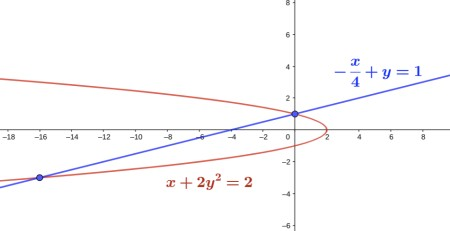# Let D be the region bounded by the parabola x + 2y^2 - 2 = 0 and the line -x/4 + y - 1 = 0. The...

## Question:

Let {eq}D {/eq} be the region bounded by the parabola {eq}x + 2y^2 - 2 = 0 {/eq} and the line {eq}- \frac{x}{4} + y - 1 = 0 {/eq}. The coordinates of the intersection points of the two curves are {eq}(-16, -3) {/eq} and {eq}(0, 1) {/eq}, and the vertex is at {eq}(2, 0) {/eq}.

A) Express {eq}D {/eq} in two types of regions.

B) Express the double integral as iterated integrals in two different orders.

C) A thin metal plate with a shape as described in {eq}D {/eq} has a density function {eq}3x^2 + 8y^2 {/eq} (pounds per unit area). Find the mass of the plate.

## Double Integrals

The integral of {eq}\displaystyle f(x,y) {/eq} over a region described horizontally-simple as {eq}\displaystyle D=\{(x,y)|\, a\leq y\leq b, u(y)\leq x\leq v(y)\} {/eq} is

{eq}\displaystyle \iint_{D}f(x,y)dA {/eq} and evaluated iteratively as {eq}\displaystyle \int_a^b\int_{u(y)}^{v(y)}f(x,y) dxdy. {/eq}

If the integrand function is challenging to integrate with respect to {eq}\displaystyle x, {/eq}

we need to change the order of integration, by describing the region vertically-simple

{eq}\displaystyle \displaystyle \mathcal{R}=\{(x,y)|\, c\leq x\leq d, w(x)\leq y\leq h(x)\} {/eq}

A) To express the region between the line {eq}\displaystyle -\frac{x}{4}+y-1=0 {/eq} and parabola {eq}\displaystyle x+2y^2-2=0 {/eq} in two different ways,

we will describe the region as a horizontally- and vertically-simple region.

The region is shown in the picture below, as being bounded by the two curves.The region is bounded at the left by the line {eq}\displaystyle x=4y-4 {/eq} and at the right by the parabola {eq}\displaystyle x=-2y^2+2, {/eq} for {eq}\displaystyle -3\leq y\leq 1, {/eq}

or {eq}\displaystyle \boxed{D=\left\{(x,y)| -3\leq y\leq 1, 4y-4\leq x\leq -2y^2+2\right\}} {/eq}

To describe the region vertically, we will split the region in

a left region, bounded above by the line {eq}\displaystyle y=1+\frac{x}{4} {/eq} and below by the part of parabola below the vertex, {eq}\displaystyle y=-\sqrt{1-\frac{x}{2}} {/eq} for {eq}\displaystyle -16\leq x\leq 0, {/eq}

and a right region, bounded above by the part of parabola above the vertex, {eq}\displaystyle y=\sqrt{1-\frac{x}{2}} {/eq} and below by the part of parabola below the vertex, {eq}\displaystyle y=-\sqrt{1-\frac{x}{2}} {/eq} for {eq}\displaystyle 0\leq x\leq 2, {/eq}

so the region is written as {eq}\displaystyle \boxed{D=\left\{(x,y)| 0\leq x\leq 2, -\sqrt{1-\frac{x}{2}}\leq y \leq \sqrt{1-\frac{x}{2}}\right\}\cup \left\{(x,y)| -16\leq x\leq 0, -\sqrt{1-\frac{x}{2}}\leq y \leq 1+\frac{x}{4} \right\}} {/eq}

B) With the two descriptions above, we can write the area of the region as the following two definite integrals.

{eq}\displaystyle \boxed{\text{Area(}D)=\int_{ -3}^1 \int_{4y-4}^{ -2y^2+2}\ dx dy}\\ \displaystyle \text{ or }\boxed{\text{Area(}D)=\int_0^2\int_{ -\sqrt{1-\frac{x}{2}}}^{\sqrt{1-\frac{x}{2}}}\ dydx+ \int_{-16}^0\int^{1+\frac{x}{4}}_{ -\sqrt{1-\frac{x}{2}}}\ dydx}. {/eq}

C) To find the mass of the plane with the shape of the region described above and density {eq}\displaystyle f(x,y)=3x^2+8y^2 {/eq}

we will use the following formula {eq}\displaystyle \text{Mass(}D)=\iint_{D}f(x,y)dA. {/eq}

Because the region is easier expressed horizontally we will use the following integral

{eq}\displaystyle \begin{align} \text{Mass(}D)&=\iint_{D}f(x,y)dA=\int_{ -3}^1 \int_{4y-4}^{ -2y^2+2} \left(3x^2+8y^2\right)\ dx dy\\ &=\int_{ -3}^1 \left(x^3+8xy^2\right)\bigg\vert_{x=4y-4}^{x=-2y^2+2}\ dy\\ &=\int_{ -3}^1 \left(x^3+8xy^2\right)\bigg\vert_{x=4y-4}^{x=-2y^2+2}\ dy\\ &=\int_{ -3}^1 \left(-8(y^2-1)^3-64(y-1)^3-16y^2(y^2+2y-3)\right)\ dy\\ &=-8\int_{ -3}^1 \left((y^2-1)^3-8(y-1)^3-2y^2(y^2+2y-3)\right)\ dy\\ &=-8\int_{ -3}^1 \left(y^6-3y^4+3y^2-1 -8(y-1)^3-2(y^4+2y^3-3y^2)\right)\ dy\\ &=-8\int_{ -3}^1 \left(y^6-5y^4+9y^2-1 -8(y-1)^3-4y^3\right)\ dy\\ &=-8\int_{ -3}^1 \left(\frac{y^7}{7}-y^5+3y^3-y -2(y-1)^4-y^4\right)\bigg\vert_{-3}^1 \\ &=\boxed{\frac{100864}{35}\approx 2881.83\ \rm pounds}. \end{align} {/eq}